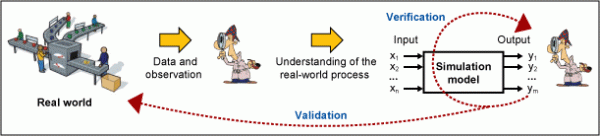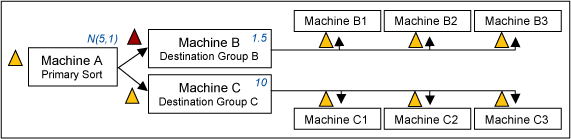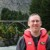# Understand the Difference Between Verification and Validation

ByWilairat Udomsawat

Many people use the terms verification and validation interchangeably without realizing the difference between the two. Not understanding that difference can lead to many models that do not truly represent a real-world process and lead to errors in forecasting or predicting of the outcomes. In this article, we will explore the meaning, importance, differences and basic method of verification and validation.

### What Is a Model?

The first step to understand these two different processes is to understand what a model is. A model, whether it is a mathematical, simulation or physical model is a representation of a real-world process. The model can be used for studying, experimenting or making a prediction of the real-world event without directly observing or making change to the real-world process.

A model is created in order to understand relationships among independent variables or inputs (Xs) and the dependent variable or the outcome (Ys). Examples of mathematical models well-known in the Lean Six Sigma (LSS) world are Little’s Law and other queuing models. Simulation models can be built using computer software. A physical model is not common to Lean applications but is frequently used for experimental purposes in engineering, architectural and science applications.

British statistician George E.P. Box said, “Essentially, all models are wrong, but some are useful,” which reminds the practitioner that neither is a model the real-world process nor can that process be fully represented. The question of how good a model can be is answered using verification and validation. The first pitfall that many LSS practitioners fall into is using the model that they created without both verifying and validating it. The second pitfall is that they go through one and assume that’s all that’s necessary. This leads to unrealistic prediction, misguided results and a loss of the integrity of the model.

### What Is Verification?

Verification is the process that ensures that the model is producing or predicting the right outcomes based on the relationships of input variables and output variables that are built into the model. The verification process does not rely on, or compare to, the real-world process. Its purpose is to confirm that the model is doing exactly what the modeler “thinks” it should do when it was created. Basically, if it is desirable for the model to return a rounded-up integer value of X1 divided by X2, does the model always provide the integer result of 1 when X1 = 3 and X2 = 4 is entered? Or does it return a result of 0.75?

Handpicked Content:   Using Monte Carlo Simulation as Process Control Aid

### What Is Validation?

Validation is the process to ensure that the model is representing the real world as much as possible. The validation process helps a modeler be certain the correct model is built. The validation process relies heavily on the data collected from the real world, and the perception and understanding of the process of the modeler. The validation process ensures that the model is doing what the real process is doing. (See Figure 1.)Figure 1: The Process of Verification and Validation

### Example: Ice Cream Stand

Consider a modeler building a model to represent a queuing system at an ice cream stand. He observes an arrival profile of customers and the service rate of the server. He finds that the server serves each customer at a constant rate of three minutes per customer. He builds a model to predict the waiting time (W) when a customer arrives at the stand and finds that there are customers (X) waiting in the system. He decides to use a mathematical model of W = 3X.

The modeler verifies that he built the model correctly by entering X = 1, 2, 5, 10 and 20 into his equation; the model returns the values of W as 3, 6, 15, 30 and 60 minutes respectively. In this verification process, the model calculates the result correctly based on the modeler’s perception of the linear relationship between W and X.

To validate this model, the modeler would conduct a time study when a customer, Jessica, arrives at the stand. For five different instances, the modeler observes there are 1, 2, 5, 10 and 20 customers in the line. The real system may return different waiting times for Jessica since some customers that are already in the line may decide to leave when the waiting time exceeds their tolerance limits. As a result, Jessica’s actual waiting time becomes shorter and thus does not consistently follow the linear relationship of W = 3X. In this case, even though the model passed the verification process, it does not represent behavior of the real system and fails the validation process.

Handpicked Content:   Turning Judgment Calls into Reliable Data with Gage R&R

### Example: Distribution Center

Why are both verification and validation of a model needed? Consider another example of a process creating a simulation model for a distribution center consisting of four product-sorting machines. In each step, a machine sorts product to its destinations. Figure 2 shows the schematic of the distribution center.Figure 2: Schematic of a Distribution Center

A LSS team collects data on cycle time and processing step at each machine. After that, the team builds a model using simulation software. Based on the data that was collected and statistically analyzed, the team found that the processing time of Machine A is normally distributed with a mean of 5 minutes and a standard deviation of 1 minute, Machine B has a constant processing time of 1.5 minutes and Machine C has a constant processing time of ten minutes. Products B and C arrive with equal distribution at Machine A every 5 minutes.

After the model was created, the team ran the model until reaching a steady state and found that there is an excessive queue in front of Machine B, but none in front of Machine C. Based on the assumption of the processing time at these three machines, and the arrival profile of products B and C, the team realizes that there could be an error in the model code or parameters. The team ensures that all parameters have been entered correctly, including breaks and lunch times, processing time and distribution types, staffing and time available in a day.

Eventually, the team found a mistake in the processing time parameter at Machine B – 15 minutes was entered instead of 1.5 minutes. This error-checking process is a verification process. By ensuring that the model is producing what it should be producing, the modeler verifies that the model is error free. Based on the assumption that Machine B sorts products faster than Machine A, there should not be any physical queue in front of it. Without a proper verification, this model would have led  to misguided results.

Handpicked Content:   Perfect Your Predictions with Yield and Single-Use Reliability Modeling

Consider the same distribution center and a corrected model. The team decides to use the model to predict the behavior of the process during a peak demand period. What is the best way to validate the model and ensure the model acts as close to the real process as possible? For an existing process for which the data is available, the process is simple. The team may use data from the previous peak period (such as work in progress, queue length and queue time from the last known period). They can use the known data as input variables and compare the results of output variables to the last known data collected to adjust the model. This way the team can ensure that the model acts similarly to the real-world process. Validating the model is not as easy when the process did not previously exist or data is not available. The team can only assume the most likely behavior of the process based on the relationships between input and output variables.

### Verification, Then Validation

The validation process should be performed after the verification process has been completed. The validation process normally involves real data, which can consume more of a team’s resources than the verification process. The table below suggests some validation methods for each modeling scenario.

 Modeling Scenarios With Corresponding Validation Methods Modeling Scenario Validation Method Model of existing process, data is available Test the model in several different cases during the normal and extreme periods using last known data and compare the model outputs to the last known outcomes Model of existing process, data is NOT available Observe behavior of real-world process and compare that to the behavior of the model Model of nonexisting process, relationships of variables are known Use correlation analysis to analyze the relationship between the outcome of the model and the input variables. Compare that to the known relationship of the variables.

### Final Thoughts

There is no one verification or validation process that fit all scenarios. A modeler should be aware of the available methods. Both verification and validation processes should be completed at the earliest stage in the project – and as thoroughly as possible. The key question for verification is whether the model was built correctly. After verification, the model should be error free. The key question for validation, on the other hand, is whether the correct model was built. After validation, it should be clear that the model acts similar to the real-world process so a team can be confident in using it to predict the behaviors of a process.

##### Wilairat Udomsawat

1.Dave Robson

Excellent article and an important one, I think.

It’s very easy to get confused between the two.

2.shantilal

Dear Wilairat
Good Day
Its nice tropic provided by you will help many to understand it clearly.
s. parmar
black belt

3.Kicab

You quote Box to state that “all models are wrong,” which I interpret as no model is correct. Thus, using the word “correct” to explain verification and validation is probably not the best choice.
In explaining validation, you state “The validation process helps a modeler be certain the correct model is built.”
In your Final Thoughts, you say “The key question for verification is whether the model was built correctly” and “The key question for validation, on the other hand, is whether the correct model was built.”
If all models are wrong, it can’t be the correct model and t’s not clear in what sense sf the model build correctly. So, you need to explain validation in other terms rather than “correct model.” Your examples provide that explanation: whether the model’s results match real-world results closely enough to be useful (per Box’s quote).

But even if a model matches real-world results it is not a correct model. There could even be several different models that match real-world results (e.g., Ptolemy’s earth-centered model’s prediction of eclipses and location of the planets vs. helio-centered model) and not all could be correct.

I like the first two parts of your table. The last case I think deviates from what you say is how to validate models. If we know the relationship among variables then there is nothing to model–unless what is known is incomplete. You still need to check your predictions against real-world results–not the variables’ relationship as that is the model.

4.Chris Seider

Nicely written….a measure of acceptability would be a good addition.

5.Bishwa

Thank you so much for the valuable insights.
I had exam of Software Engineering yesterday!
It was just the information I was looking for.
keep going :)
Thanks :)